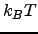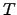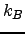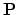Next: Graph Theory Representation of Up: ENSEMBLES OF REARRANGEMENT PATHWAYS Previous: ENSEMBLES OF REARRANGEMENT PATHWAYS   Contents

# Introduction

Stochastic processes are widely used to treat phenomena with random factors and noise. Markov processes are an important class of stochastic processes for which future transitions do not depend upon how the current state was reached. Markov processes restricted to a discrete, finite, or countably infinite state space are called Markov chains [188,187,123]. The parameter that is used to number all the states in the state space is called the time parameter. Many interesting problems of chemical kinetics concern the analysis of finite-state samples of otherwise infinite state space .

When analysing the kinetic databases obtained from discrete path sampling (DPS) studies  it can be difficult to extract the phenomenological rate constants for processes that occur over very long time scales . DPS databases are composed of local minima of the potential energy surface (PES) and the transition states that connect them. While minima correspond to mechanically stable structures, the transition states specify how these structures interconvert and the corresponding rates. Whenever the potential energy barrier for the event of interest is large in comparison withthe event becomes rare, whereis the temperature andis Boltzmann's constant.

The most important tools previously employed to extract kinetic information from a DPS stationary point database are the master equation , kinetic Monte Carlo [190,191] (KMC) and matrix multiplication (MM) methods . The system of linear master equations in its matrix formulation can be solved numerically to yield the time evolution of the occupation probabilities starting from an arbitrary initial distribution. This approach works well only for small problems, as the diagonalisation of the transition matrix,, scales as the cube of the number of states . In addition, numerical problems arise when the magnitude of the eigenvalues corresponding to the slowest relaxation modes approaches the precision of the zero eigenvalue corresponding to equilibrium . The KMC approach is a stochastic technique that is commonly used to simulate the dynamics of various physical and chemical systems, examples being formation of crystal structures , nanoparticle growth  and diffusion . The MM approach provides a way to sum contributions to phenomenological two-state rate constants from pathways that contain progressively more steps. It is based upon a steady-state approximation, and provides the corresponding solution to the linear master equation [196,189]. The MM approach has been used to analyse DPS databases in a number of systems ranging from Lennard-Jones clusters [10,8] to biomolecules [133,197].

Both the standard KMC and MM formulations provide rates at a computational cost that generally grows exponentially as the temperature is decreased. In this chapter we describe alternative methods that are deterministic and formally exact, where the computational requirements are independent of the temperature and the time scale on which the process of interest takes place.

SubsectionsNext: Graph Theory Representation of Up: ENSEMBLES OF REARRANGEMENT PATHWAYS Previous: ENSEMBLES OF REARRANGEMENT PATHWAYS   Contents
Semen A Trygubenko 2006-04-10